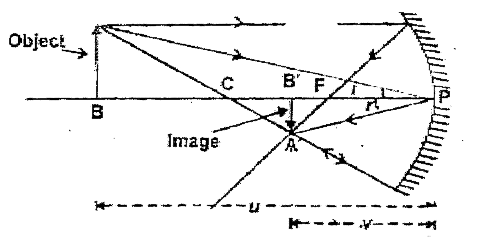Question

Updated on:30/05/2023

# Derive mirror formula for a convex mirror.

Text Solution

Solution
New Cartesian sign conventions :
(i) All the distances are measured from the pole of the mirror.
(ii) All the distances measured in the direction of incident ray are taken as positive and those opposite to the direction of incident ray are taken as negative.
(iii) All distances measured in vertically upward direction from the principal axis are taken as positive and in vertically downward direction as negative.
Mirror formula A relation between distance of object, distance of image from mirror and focal length of the mirror is known as mirror formula.
Derivation of mirror formula : Let an object AB is held perpendicular to principal axis of concave mirror beyond C and it forms the real and inverted image A.B between C and F as shown in Fig:As triangles ABC and A.BC axe similar,
ABA.B.=CBCB ....(1)
Again triangles ABPand A.BP are similar,
ABA.B.=PBPB.....(2)
From eqns. (1) and (2), we have
CBCB=PBPB ..(3)
Measuring all distances from P, we have
CB=PBPC
CB.=PCPB.
From eq. (3),
PBPCPCPB=PBPB
Using new Cartesian sign conventions, we have PB = -U (Distance of object measured against incident light) PC= - R"(Radius of curvature measured against incident light) PB =-v (Distance of. image measured against incident light) We get from eqn. (4)
We get from eqn. (4)
u(R)R(v)=uv or u+RR+v=uv
Cross multiplying
vuvR=uRuv or uR+vR=2uv...(5)
Dividing both sides by uvR, we get
uRuvR+vRuvR=2uv4uvR
1v+1u=2R
But we know that R = 2f
1u+1v=22f
1u+1v=1f
which is the required mirror formula.